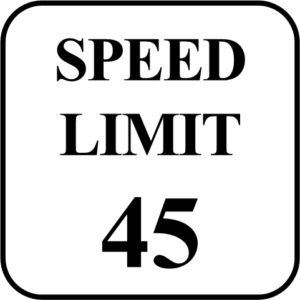# Physical Quantities and Units | Systems of Units

Want create site? Find Free WordPress Themes and plugins.

Physical quantities are values determined by making measurements or obtained by being calculated from other measurements.  An example of obtaining a value from a measurement is using an odometer or another measurement device to determine the distance a car travels or using a watch to determine how much time it took to make the trip.  The average speed the car travels during the trip can be calculated by dividing the distance by the time, values that were obtained from measurement devices.Fig.1: Speed limits Determined by Rate and Distance

## Systems of Units

When making measurements, physical quantities are typically expressed in standardized units.  For example, grams are used to indicate the weight of an object or substance that is relatively small, and kilograms indicate the weight of something that is much heavier.  There are two major standardized systems indicating units that are used throughout the world, English units and SI units.  English units are used in the United States and use pounds to indicate weight, and inches, feet, yards, and miles to indicate length and distance.  SI units, which is also known as the metric system, is used in most other parts of the world to make measurements.  The metric system is also used by the scientific community when making measurements or to indicate quantitative values.

## SI Units: Fundamental and Derived Units

The basic laws of physics are typically described in terms of quantities resulting from measurements taken while performing experiments, when making calculations, or from information obtained when a simulation is performed.  These quantities are expressed in units.  These quantities are referred to as fundamental units. Fundamental units of measure include meters to indicate distance, kilograms to indicate weight and seconds to represent time.  There are other physical quantities which are expressed as a combination of two or more units of measurement.  For example, the average speed of a car traveling in Europe is determined by dividing distance (or length) in kilometers by time in hours. The average speed is an example of a quantity referred to as derived units.

## Units of Time, Length, And Mass: The Second, Meter, And Kilogram

To communicate the result of a measurement of a physical quantity, a unit for the quantity needs to be defined.  For example, the fundamental SI unit of time is the second which is given the abbreviation (s).  For length, the SI fundamental unit is the meter which is given the abbreviation (m).  The SI fundamental unit for mass is the kilogram, which is given the abbreviation (kg).

## Metric Prefixes

In physics, very large and very small numbers with many digits are often used. To make it manageable to work with these number when making calculations or simply writing a value, a variety of methods are used. One example is Scientific notation, which is a method that uses a coefficient value between 1 and 10, and powers of 10 that represent a value based on an exponent number. Another variation of Scientific notation is called Engineering notation, which uses a power of 10 in multiples of three.  Large and small numbers are also simplified and reduced in size by Metric prefixes that use prefixes that have 4 or 5 letters of the alphabet.  These multiple letters can be designated by Greek or English letters.  The following table shows the most common metric prefixes used to represent physics related values, and how they pertain to Engineering notation and whole number values.

 Metric Prefix Whole Number Engineering Notation Value Giga (G) 1,000,000,000 1012 one billion Mega (M) 1,000,000 106 one million Kilo (K) 1,000 103 one thousand milli (m) 0.001 10-3 one-thousandth micro (μ) 0.000001 10-6 one-millionth nano (n) 0.000000001 10-9 one-billionth

## Unit Conversion and Dimensional Analysis

When converting a metric quantity to or from a whole number, it is necessary to move the decimal point to the right or to the left.  The direction is dependent on the type of conversion made.

The following rules apply to metric quantity conversions.

1. When converting from a larger value to a smaller value, move the decimal point to the right.
2. When converting from a smaller value to a larger value, move the decimal point to the left.
3. Determine the number of places the decimal point needs to be moved by finding the difference between the base value and the specific metric unit.

Review Questions

1.  Physical quantities are values determined _______.

• by making measurements
• from calculations of readings measured by instruments
• Both a and b

2. English units indicate values using the metric system. T/F

3. If the posted speed limit on a Canadian highway is 100km/h, what is this in meters per second?

• 1660m/s
• 166m/s
• 8m/s
• 278m/s

4. The SI fundamental unit for mass is the ____________.

5. The engineering notation for the metric prefix Giga is _____.

• 106
• 109
• 1012
• 1015

6. The whole number for 10-3 is _________.

7. The whole number for one-millionth is ______.

• 001
• 000001
• 000000001

8. When converting a metric quantity from a larger value to a smaller value, move the decimal point to the _________.